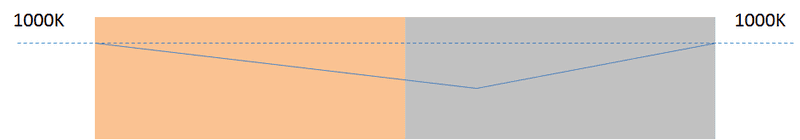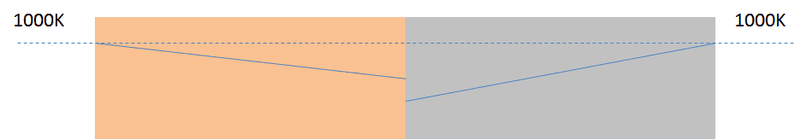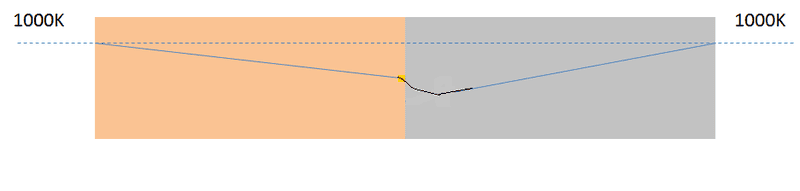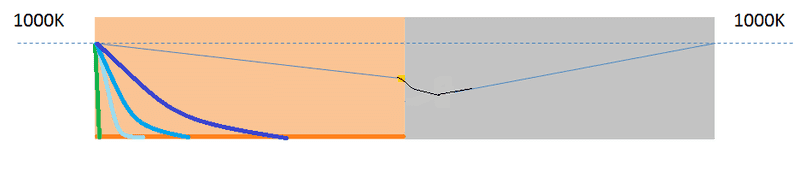# Temperature Profile -- temperature-length curve of a metal bar

Guys...
If there is a bar, half of it is Copper and another half is Steel (Length wise) and both of its ends are at 1000K and I want to know how to find the temperature- length curve.
this was an interview Question by the way.

Hi, Kishore. I would use Fourier's heat equation to model each half of the bar, simplify according to the physical situation, then solve each equation using the appropiate boundary conditions.

You need an equation for each half because the thermal diffusivities of the materials are different, so each half will have a different temperature profile, even if both ends are at 1000 K. See chapters 10 and 11 of Transport Phenomena by Bird et al.

Hi, Kishore. I would use Fourier's heat equation to model each half of the bar, simplify according to the physical situation, then solve each equation using the appropiate boundary conditions.

You need an equation for each half because the thermal diffusivities of the materials are different, so each half will have a different temperature profile, even if both ends are at 1000 K. See chapters 10 and 11 of Transport Phenomena by Bird et al.
So.....How to find the temperature at the junction?
Is it constant or Varies with time?

Chestermiller
Mentor
So.....How to find the temperature at the junction?
Is it constant or Varies with time?
The boundary condition at the junction is that the heat flux is constant across the junction.

Guys...
If there is a bar, half of it is Copper and another half is Steel (Length wise) and both of its ends are at 1000K and I want to know how to find the temperature- length curve.
this was an interview Question by the way.
Is the curve required in the equilibrium state or at some arbitrary time, before equilibrium is reached?
I think that for an interview, it should be the equilibrium case.
And what are the lateral boundary conditions? Isolated bar?

Is the curve required in the equilibrium state or at some arbitrary time, before equilibrium is reached?
I think that for an interview, it should be the equilibrium case.
And what are the lateral boundary conditions? Isolated bar?
Yep...it's an isolated bar...

Then you don't need any equations.

•Nidum
Then you don't need any equations.
Then how to find temperature at the junction?

What will be the temperature at equilibrium? You have an object in contact with a source at 1000 degrees. No heat is dissipated, all is used to increase the temperature of the object. When will the heat transfer stop?

Khashishi
Who said no heat is dissipated? That would make this a rather trivial problem.

If both ends are at 1000K there is no heat flow along the bar.
Is this a trick question or have I misunderstood your statement?

Who said no heat is dissipated? That would make this a rather trivial problem.
What would you understand from "the bar is isolated"?
And yes, in this case is quite simple.

The ends may be at 1000 while the rest of the bar is not. Yet.

What would you understand from "the bar is isolated"?
And yes, in this case is quite simple.

The ends may be at 1000 while the rest of the bar is not. Yet.

Guys...I don't know the complete question.
Some guy posted this question.(Not completely)
I asked u that question becoz I am also preparing for the same interview.
What do u guys think the correct question could be? I mean like the boundary conditions etc...

Nidum
Gold Member
Guys...
If there is a bar, half of it is Copper and another half is Steel (Length wise) and both of its ends are at 1000K and I want to know how to find the temperature- length curve.
this was an interview Question by the way.

This is a standard type of interview question . The interviewer is just trying to find out if you know anything about the subject and more generally if you can solve technical problems .

I was once asked essentially the same question but relating to engine components rather than a plain bar .

Possible responses :

(b) Give a descriptive answer saying in words what you think the temperature distribution would be under different conditions .

(c) Sketch a reasonable approximation to the actual temperature curve based on dimensions and boundary conditions which you propose yourself .

When going to any technical interview find out beforehand what subjects the people interviewing you are likely to be interested in .

•lychette
Staff Emeritus
2021 Award
I asked u that question becoz
1. Please use proper English and not text speak.
2. How do you expect us to know the answer if you don't know the question?

•gmax137Left side is copper bar and right side is steel. Neglecting the effect of thermal contact reisistance; If you consider this then diagram should be like below;Above diagram is not an equilibrium diagram; equilibrium diagram would be simply horizontal line of 1000K.
As thermal conductivity of both the bars are different that means slope of temperature profile would be different inside bars.
Please correct me if I am wrong, #Mentors

I ag
This is a standard type of interview question . The interviewer is just trying to find out if you know anything about the subject and more generally if you can solve technical problems .

I was once asked essentially the same question but relating to engine components rather than a plain bar .

Possible responses :

(b) Give a descriptive answer saying in words what you think the temperature distribution would be under different conditions .

(c) Sketch a reasonable approximation to the actual temperature curve based on dimensions and boundary conditions which you propose yourself .

When going to any technical interview find out beforehand what subjects the people interviewing you are likely to be interested in .
I agree...it is an open ended question to start discussion. I doubt there is a 'correct' answer

256bits
Gold Member
View attachment 101776
Left side is copper bar and right side is steel. Neglecting the effect of thermal contact reisistance; If you consider this then diagram should be like below;
View attachment 101777
Above diagram is not an equilibrium diagram; equilibrium diagram would be simply horizontal line of 1000K.
As thermal conductivity of both the bars are different that means slope of temperature profile would be different inside bars.
Please correct me if I am wrong, #Mentors

Assuming a radially insulated bar - no heat flow in the y or z direction - heat flow only along the x-axis.
Assuming heat conduction of copper > heat conduction of steel.
assuming heat capacity of copper is < heat capacity of steel.

You do not give any time frame for your diagrams.
Nor a pick for the starting temperature of the bar(s).

If we take the top diagram, and have the initial temperature, T0, of the bar(s) at time t0.
If we assume T0 at the bottom of the graph, then the temperature profile is what it would be 3/4 ( approxiametely, could be 7/8, but more than half way through to equilibrium ) of the time it would take the bar(s) to reach 1000 C throughout.

And NOT at to when the bars have the 1000 C applied to their ends.

The line from the left to the right, should have one slope for the copper, and a different slope for the steel, as the thermal conductivity and the thermal diffusivity for each are not the same.

The one correct thing is that the two lines left to right, and right to left, I would agree, should meet at the same temperature somewhere in the steel.

The bottom graph should have the temperatures profiles from the left and from the right meeting somewhere also in the steel. What you have is the heat flow abruptly stopping at the copper-steel junction. Not sure why - Did you put for some reason a perfect insulator there? Or a good choice you made that the contact resistance >> conductivity resistance?

********************************
You should take note that there is such as thing as heat capacity as well as heat conduction. Both are properties of material. If we slice our hypothetical bar into sections, then before at any section of a material can conduct heat to the next section, it has to absorb heat. By absorbing heat, the temperature of the section will rise and only then can heat be sent along to the next section. Of course this does not happen in discrete steps but is a continious process, with the sections of width dx, and temperature between sections of dT.

At the start of applying the 1000 C, there is a large temperature difference from that to the first section, the slope dT/dx should be very steep. As time progresses, the slope should become more and more horizontal, until as you say, at equilibrium, the whole bar is at one temperature of 1000 C.

Having said that, at no time though, as time passes, is the temperature profile an exactly completely straight line from the 1000 C through to the sections of bar that have already been heated up to the particular section of the bar that is just beginning to heat up, ( at a lower dT/dx than precious sections ) , wherever that particular section be may be.

******************************************
Problem:
What if we assume:
heat conduction of copper > heat conduction of steel
but
heat capacity of copper > heat capacity of steel

**************************************
At the interview you may get a similar but not the same question.
- if the copper length is twice the length of the steel.

Your answer at the interview has to be flexible yet brief enough to show your knowledge on the subject matter at hand.
related terms:
heat conduction
heat capacity
thermal diffusivity.
general heat conduction equation
http://booksite.elsevier.com/samplechapters/9780123735881/9780123735881.pdf section 1.3 Page 6, Equation 1.12 page 7, Eq 1.29 Page 2
( Note that the equation is parabolic and not straight line )
see graph Fg 1.6 Note the logrithmic scaling of temperature

Good luck reinforcing your familiarity with the subject matter.

•Ravi Singh choudhary
Chestermiller
Mentor
I think the main thing that @256bits is saying (please correct me if I am wrong) is that the heat conduction situation within the bar might be transient, meaning that the temperature is changing as a function of time. If this is the case then, no matter what the initial temperature profile within the bar was, at all times greater than time zero, the temperature profile will be continuous. And the required boundary condition at the interface between the two materials will be that the heat flux is continuous across the interface. So, the two temperatures match at the interface, and the two heat fluxes will match at the interface.

Chet

256bits
Gold Member
I think the main thing that @256bits is saying (please correct me if I am wrong) is that the heat conduction situation within the bar might be transient, meaning that the temperature is changing as a function of time. If this is the case then, no matter what the initial temperature profile within the bar was, at all times greater than time zero, the temperature profile will be continuous. And the required boundary condition at the interface between the two materials will be that the heat flux is continuous across the interface. So, the two temperatures match at the interface, and the two heat fluxes will match at the interface.

Chet
Yes , a condensed summary, of a transient heat flow.

I think one have an incorrect statement, ( uneditable ).
If we assume T0 at the bottom of the graph, then the temperature profile is what it would be 1/4 ( approximately, could be 1/8, but less than half way through to equilibrium ) of the time it would take the bar(s) to reach 1000 C throughout.

If we take the top diagram, and have the initial temperature, T0, of the bar(s) at time t0.
If we assume T0 at the bottom of the graph, then the temperature profile is what it would be 3/4 ( approxiametely, could be 7/8, but more than half way through to equilibrium ) of the time it would take the bar(s) to reach 1000 C throughout.

What do you mean by 3/4 and 7/8 here?

The line from the left to the right, should have one slope for the copper, and a different slope for the steel, as the thermal conductivity and the thermal diffusivity for each are not the same.

I have drawn different slopes for them; as diameter of bars are small, it appears to be same almost. but as length is same for both the bars and they are not meeting at centre, that means they have different slopes.

The bottom graph should have the temperatures profiles from the left and from the right meeting somewhere also in the steel. What you have is the heat flow abruptly stopping at the copper-steel junction. Not sure why - Did you put for some reason a perfect insulator there? Or a good choice you made that the contact resistance >> conductivity resistance?

I admit that I made a mistake; it should not be abrupt. It should be like belowYou should take note that there is such as thing as heat capacity as well as heat conduction. Both are properties of material. If we slice our hypothetical bar into sections, then before at any section of a material can conduct heat to the next section, it has to absorb heat. By absorbing heat, the temperature of the section will rise and only then can heat be sent along to the next section. Of course this does not happen in discrete steps but is a continious process, with the sections of width dx, and temperature between sections of dT.

As in the steady state; the transient term vanishes so the heat capacity term. That means heat capacity has role in the changing of slope with time, but originally at t=0 which one of the two slope will be steeper will be defined by the conductivity only.

One more this I have doubt about thermal diffusivity, how we compare the two thermal diffusivity; I know the mathematical definition. But I need some more insight to this.

Thanks for such an elaboration.

256bits
Gold Member
What do you mean by 3/4 and 7/8 here?
See the edit I did .I admit it is not a particularly well formed statement, and neither is the edit.

As you know, difference in temperature drives the heat flow.
As the temperature of the bar(s) increases, generally speaking the dT/dx becomes increasingly smaller. Thus as time goes by less and less heat flows into the bar(s).
The time for the left and right heat flows to meet at a halfway temperature between initial temperature and the final temperature ( 1000 C ) is less than the time to go from that halfway temperature to the final temperature.

I admit that I made a mistake; it should not be abrupt. It should be like below
Not necessarily a mistake. If the bars are loosely thermally connected, your graph could very well be more correct than not.

As in the steady state; the transient term vanishes so the heat capacity term. That means heat capacity has role in the changing of slope with time, but originally at t=0 which one of the two slope will be steeper will be defined by the conductivity only.
I think that is correct.
For this bar case, steady state is reached only when the whole bar is at 1000 c.

One more this I have doubt about thermal diffusivity, how we compare the two thermal diffusivity; I know the mathematical definition. But I need some more insight to this.
It is a term that shows how quickly the heat front moves through the material.
If I can phrase it this way,
The thermal conduction wants the heat to keep on moving forward.
The thermal capacity wants to keep the heat to stay where it is.

I have drawn different slopes for them; as diameter of bars are small, it appears to be same almost. but as length is same for both the bars and they are not meeting at centre, that means they have different slopes.
You drew a little curve there which is how it should look. Note that the lines from the ends of the bars which appear to be straight, also have some curve, barely noticeable due to the, as you say, the scale of the graph from the thinness of the bars.

•Ravi Singh choudhary
256bits
Gold Member
I have drawn different slopes for them; as diameter of bars are small, it appears to be same almost. but as length is same for both the bars and they are not meeting at centre, that means they have different slopes.

Your transient temperature profile should look something like this, as time goes by,
I have shown only the copper side.Orange - initial temperature of the bar.
Green - an instant after the 1000 C is applied
Light Blue - At some time t1
Mid Blue- at some time t2
Blue - at some time t3

•Ravi Singh choudhary
I think OP has got his answer. He should verify it.